# NCERT Solutions Class 5 Maths Chapter 13 Ways To Multiply And Divide

Here you can get free NCERT Solutions for Class 5 Maths, of Chapter 13 Ways To Multiply And Divide. All NCERT Book Solutions are given here exercise wise for Ways To Multiply And Divide. NCERT Solutions are helpful in the preparation of several school level, graduate and undergraduate level competitive exams. Practicing questions from NCERT Maths Book, its Solutions for Class 5 Chapter 13 Ways To Multiply And Divide is proven to enhance your subject skills.

 Class: 5th Class Chapter: Chapter 13 Name: Ways To Multiply And Divide Subject: Maths

## NCERT Solutions Class 5 Maths Chapter 13 – Ways To Multiply And Divide

NCERT Class 5 Solutions Chapter 13 – Ways To Multiply And Divide are given below.

NCERT Solutions for Class 5 Maths (Math Magic) Chapter 13 Ways to Multiply and Divide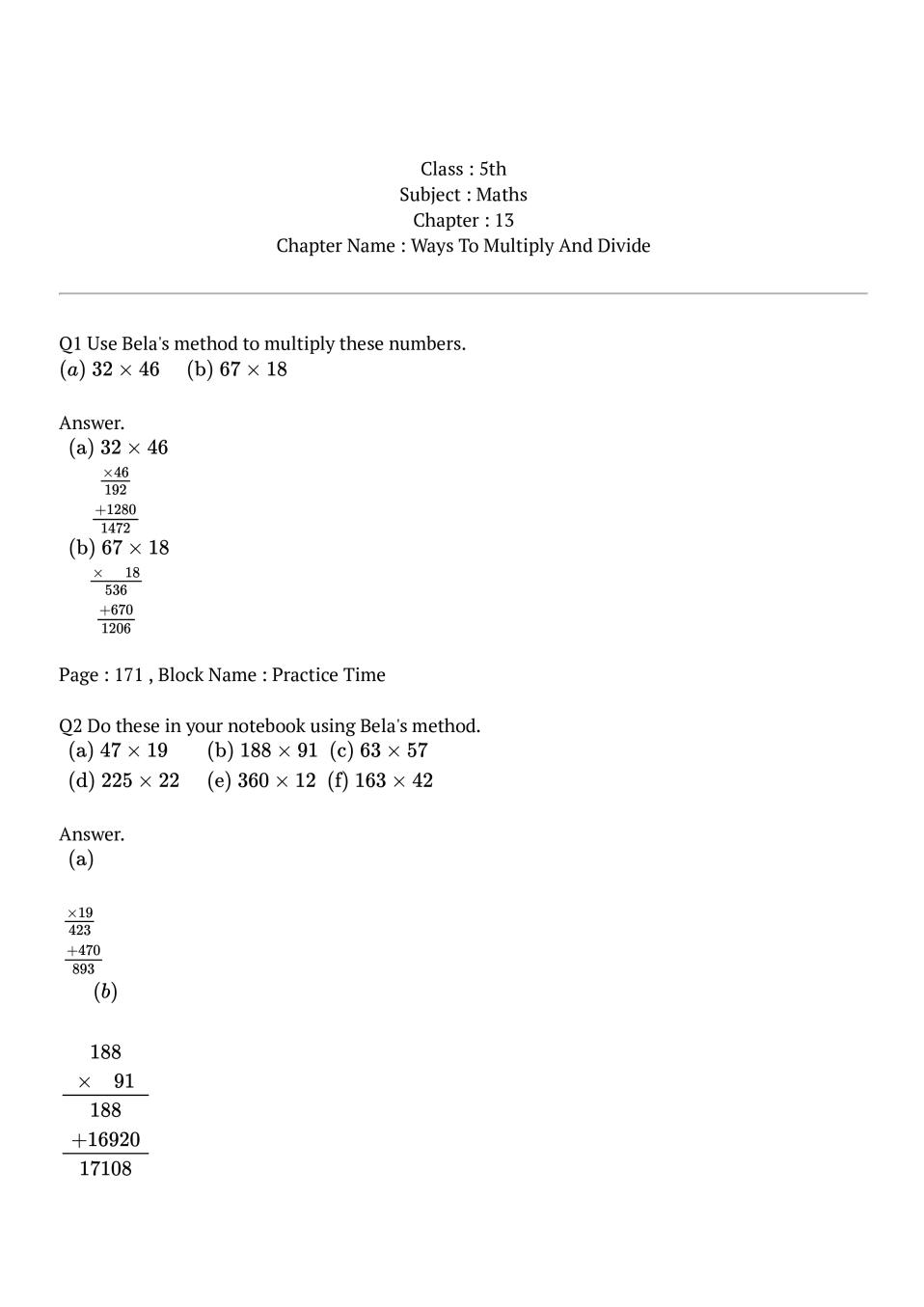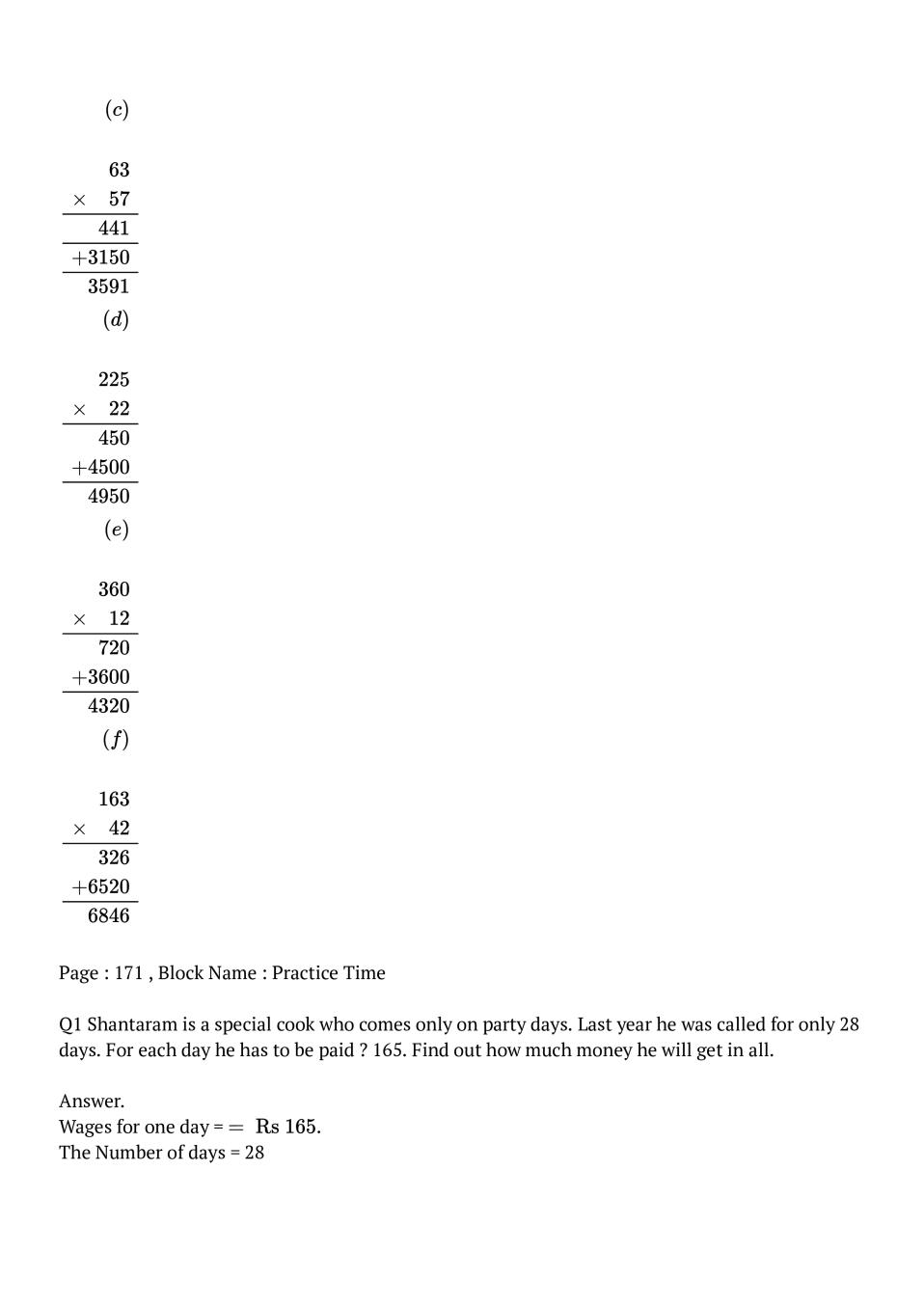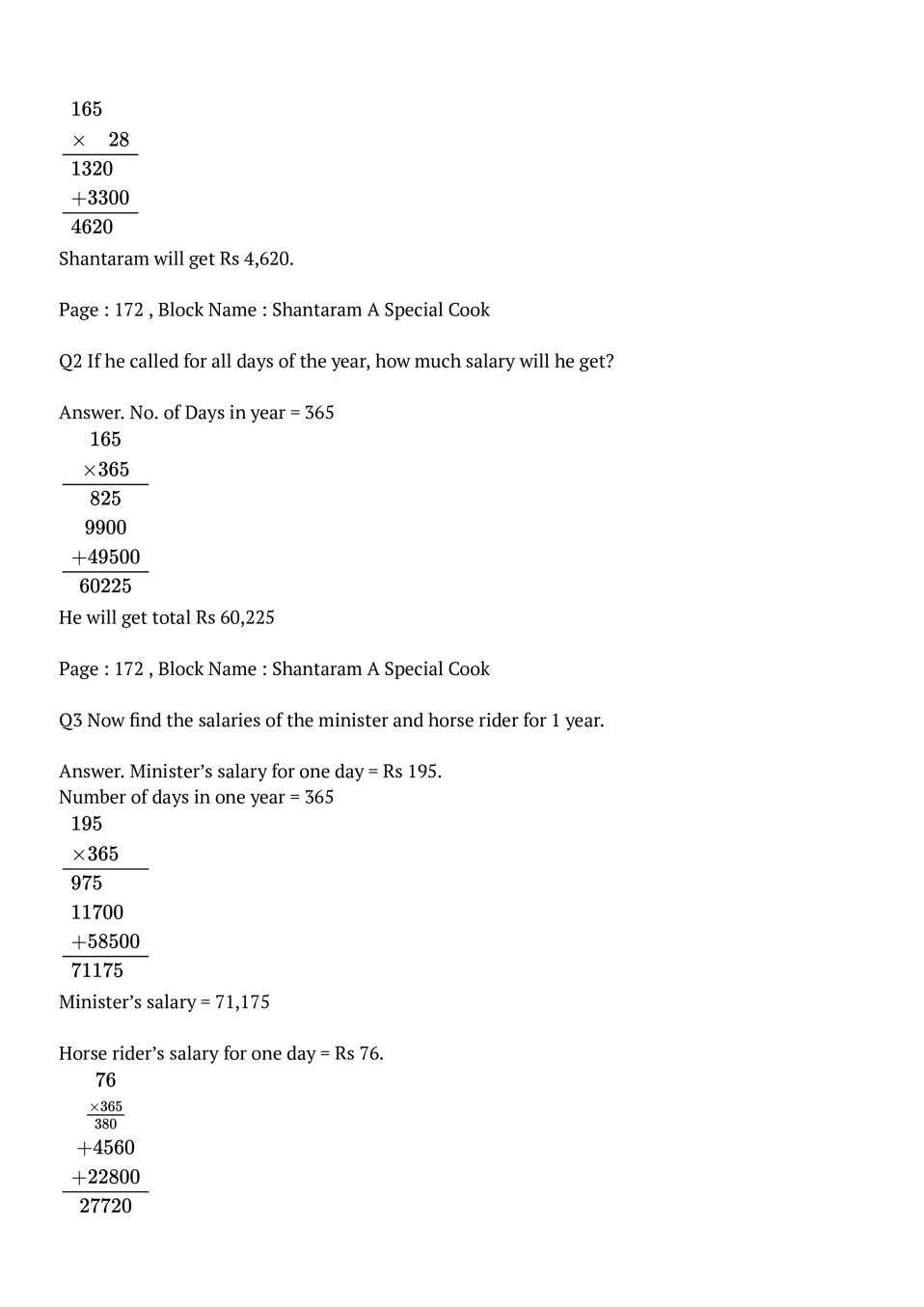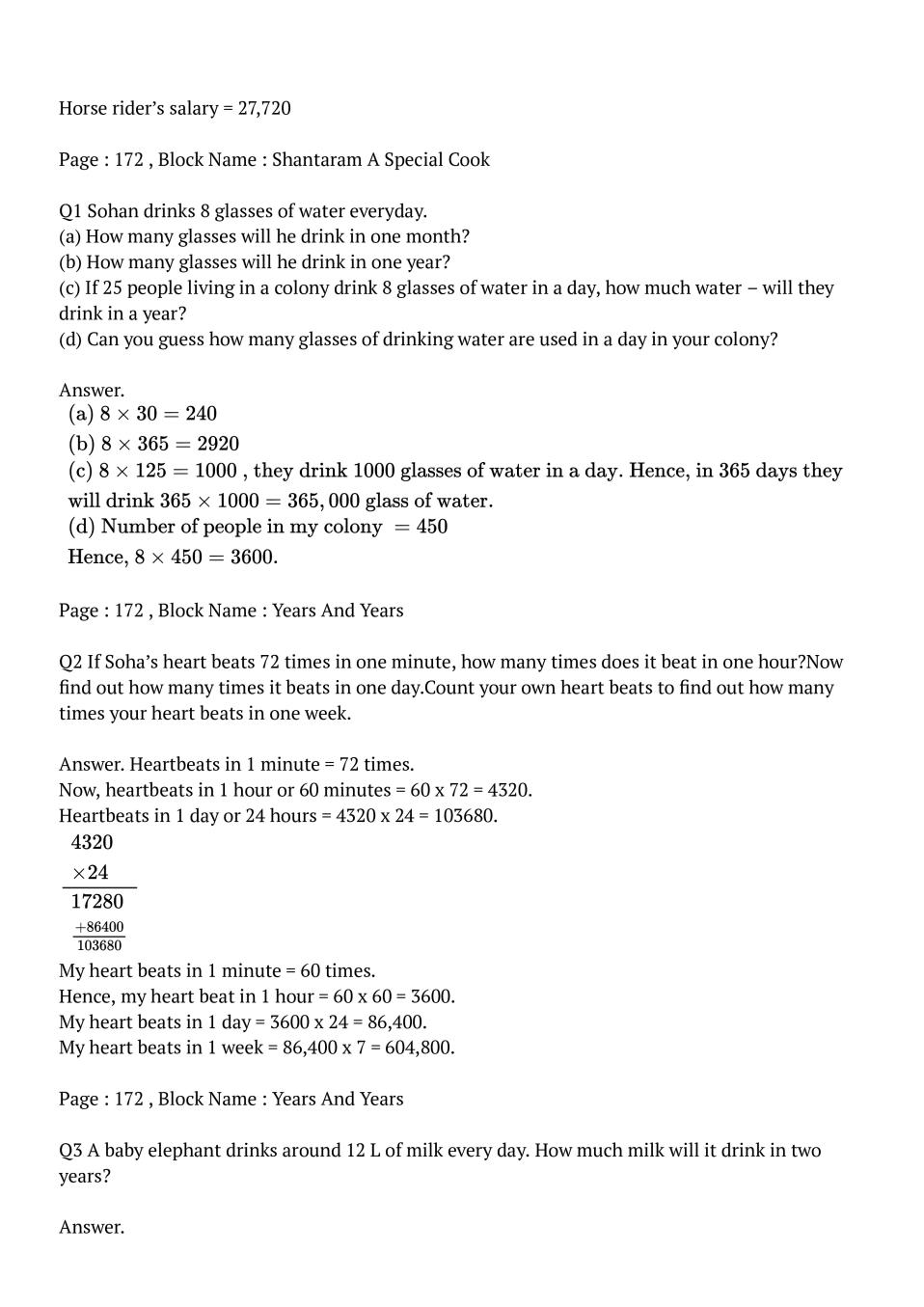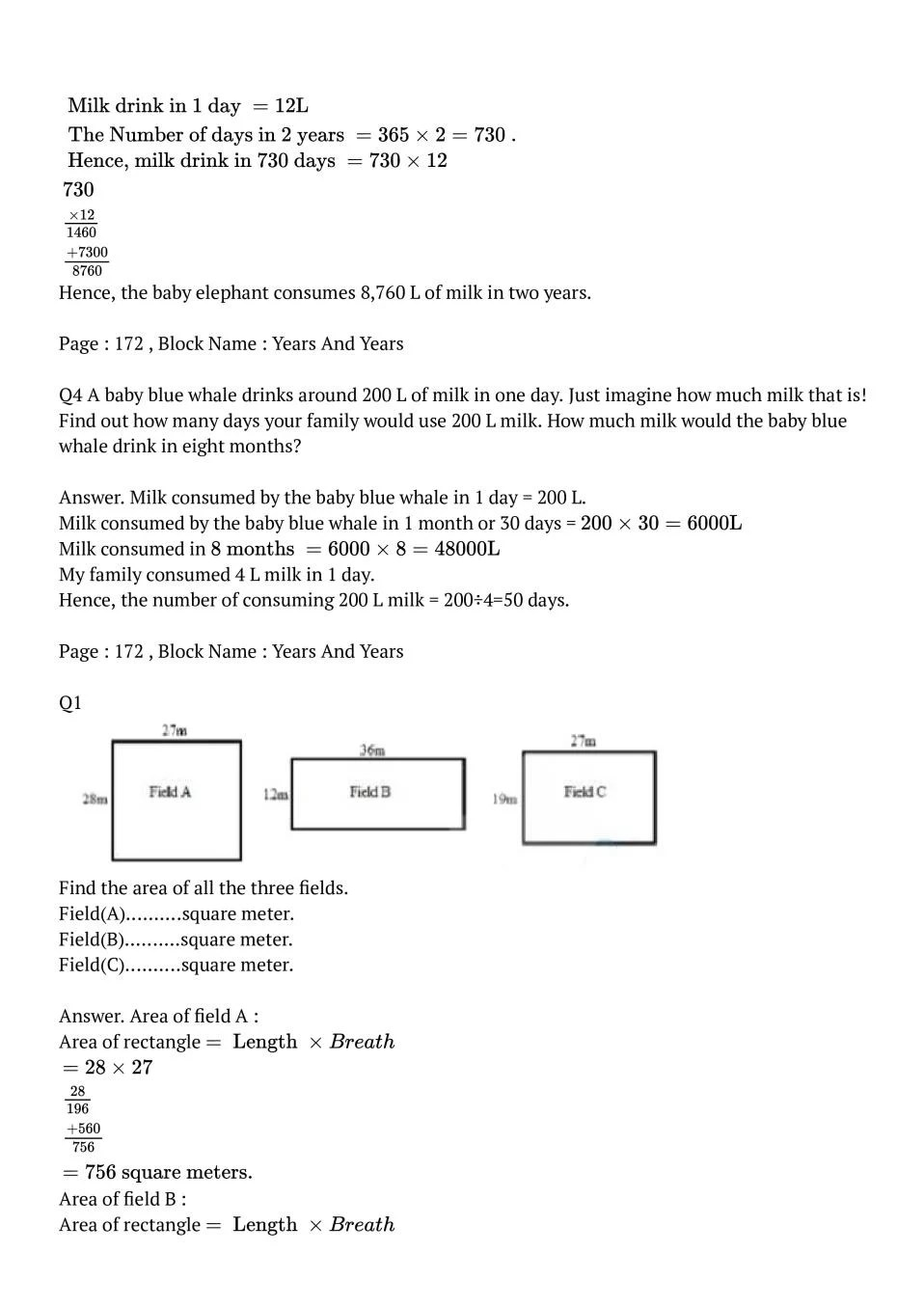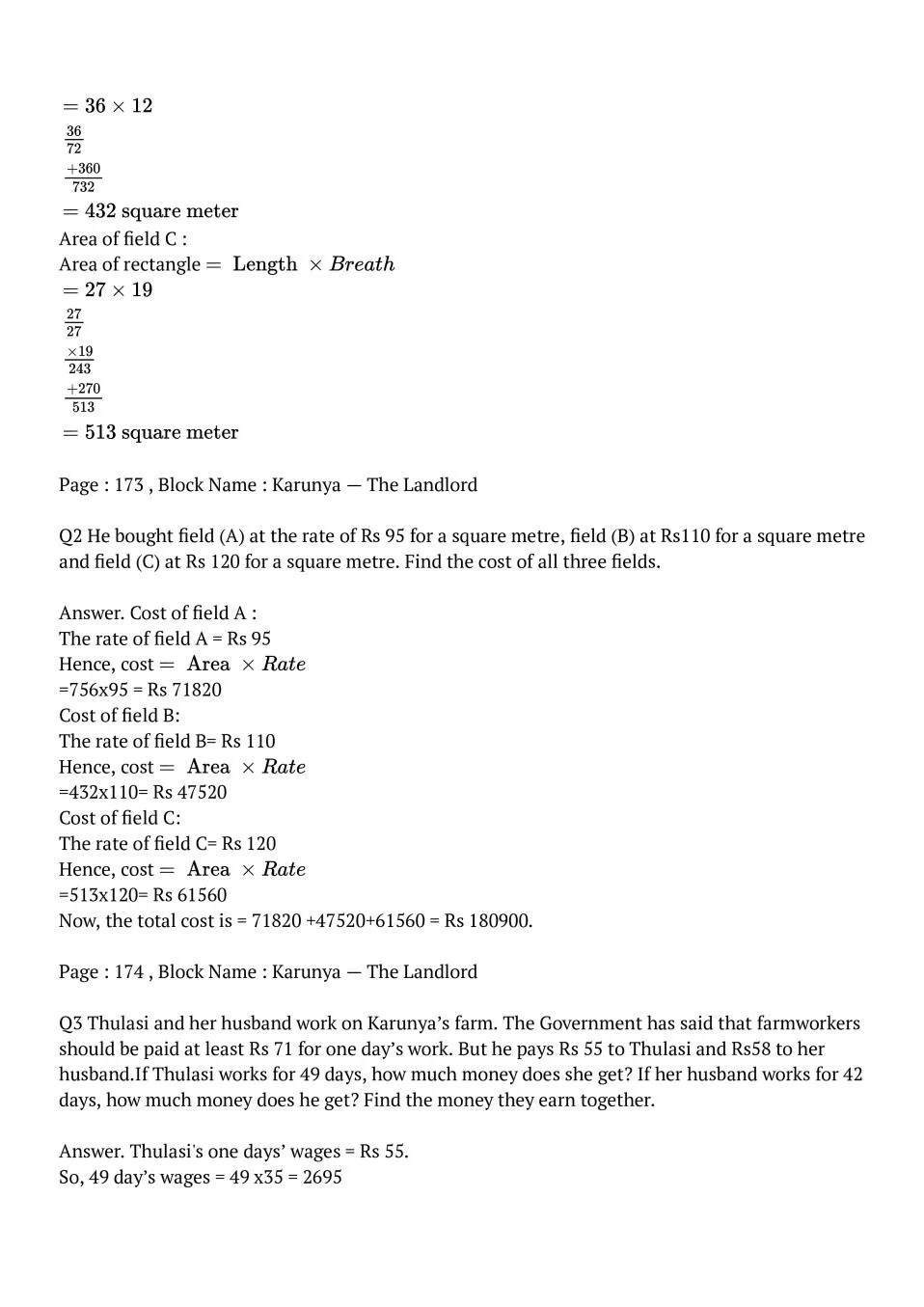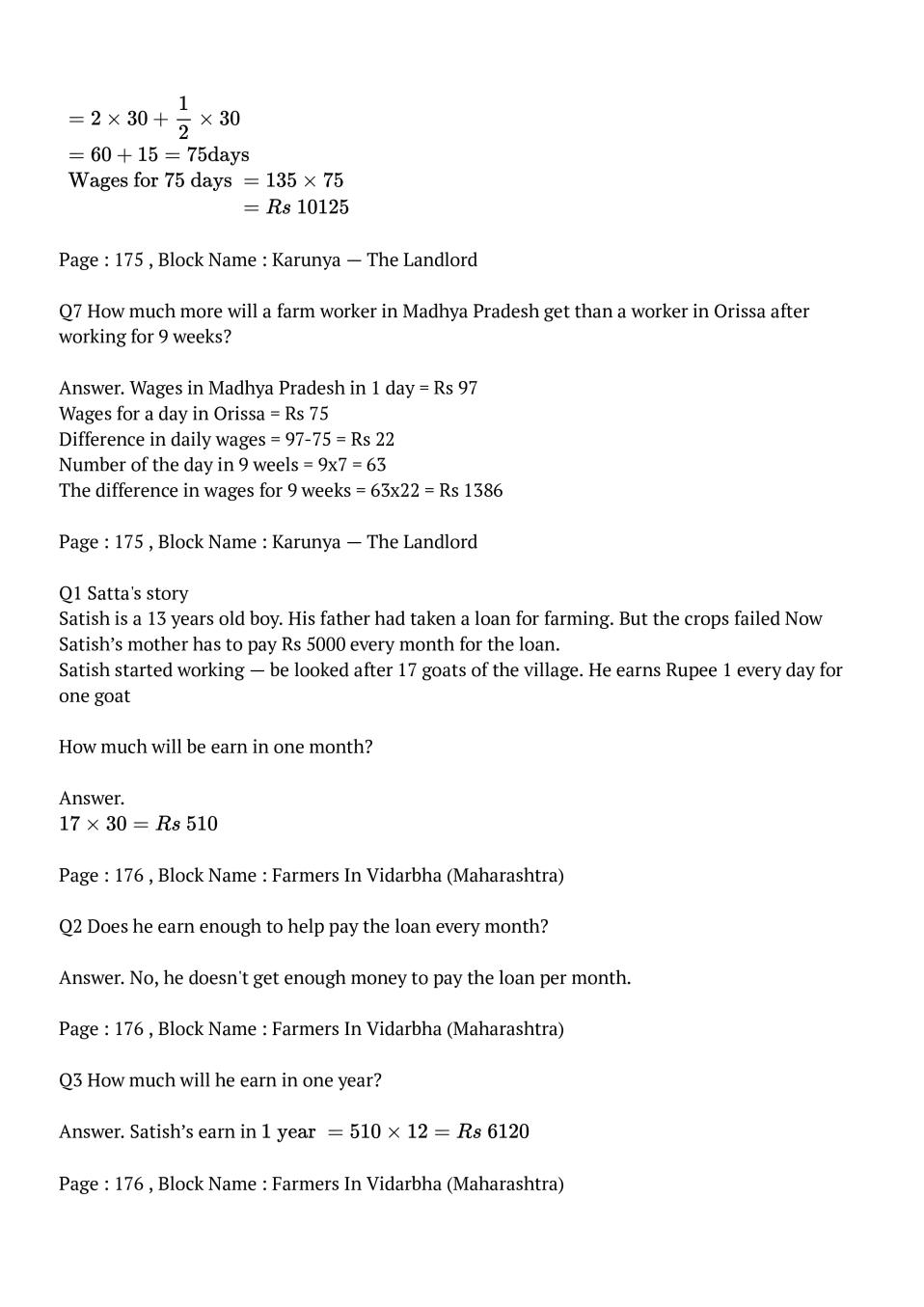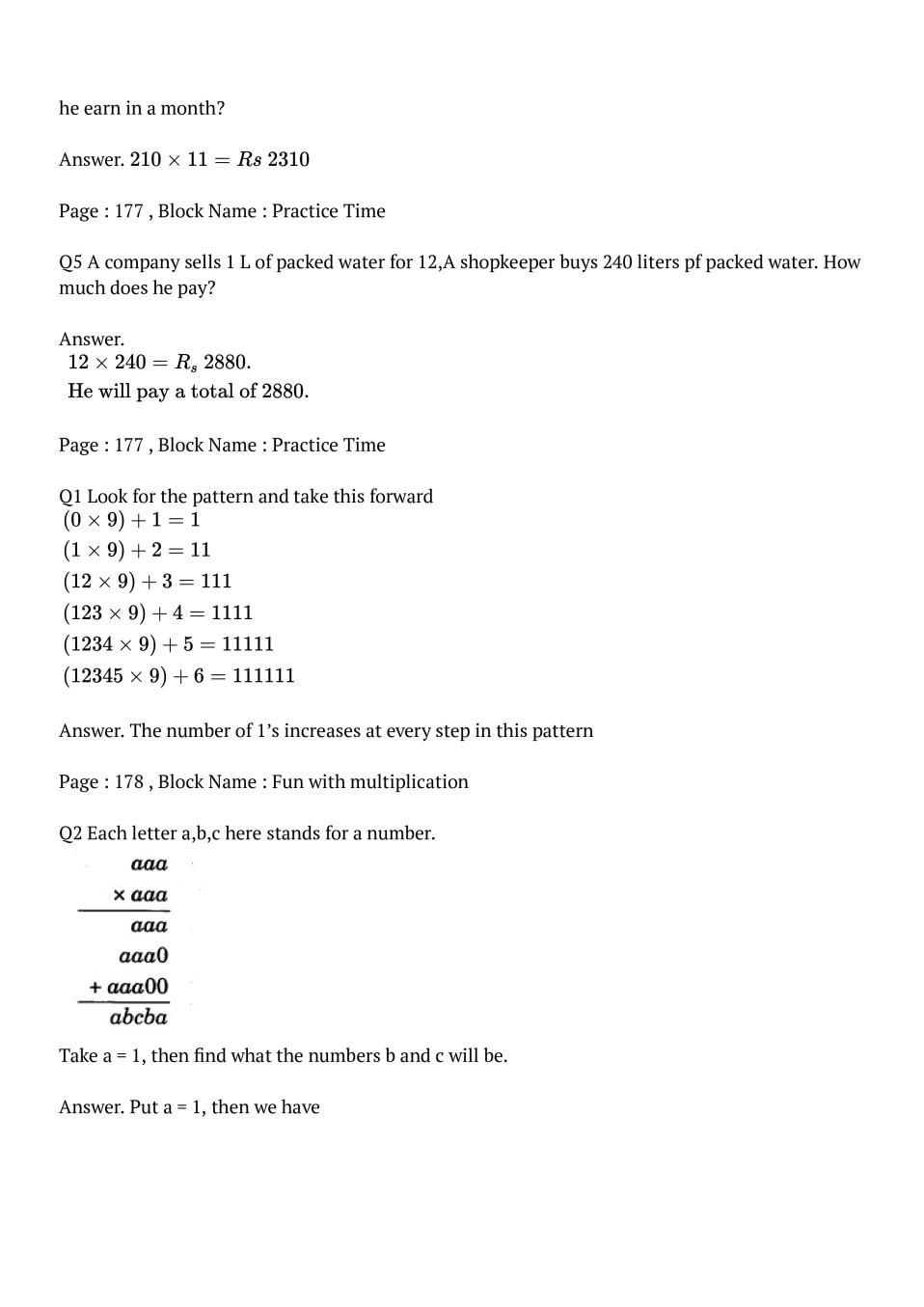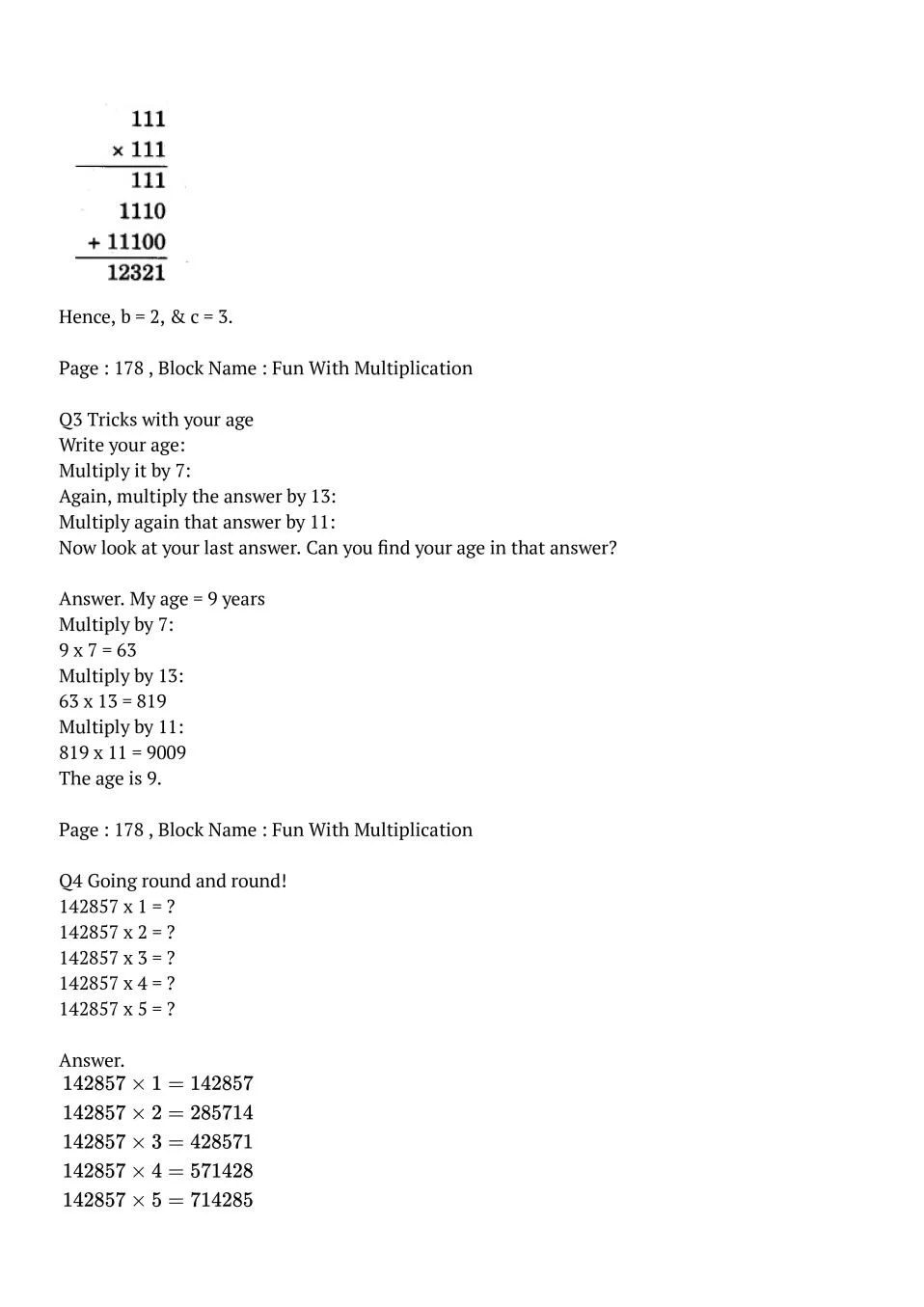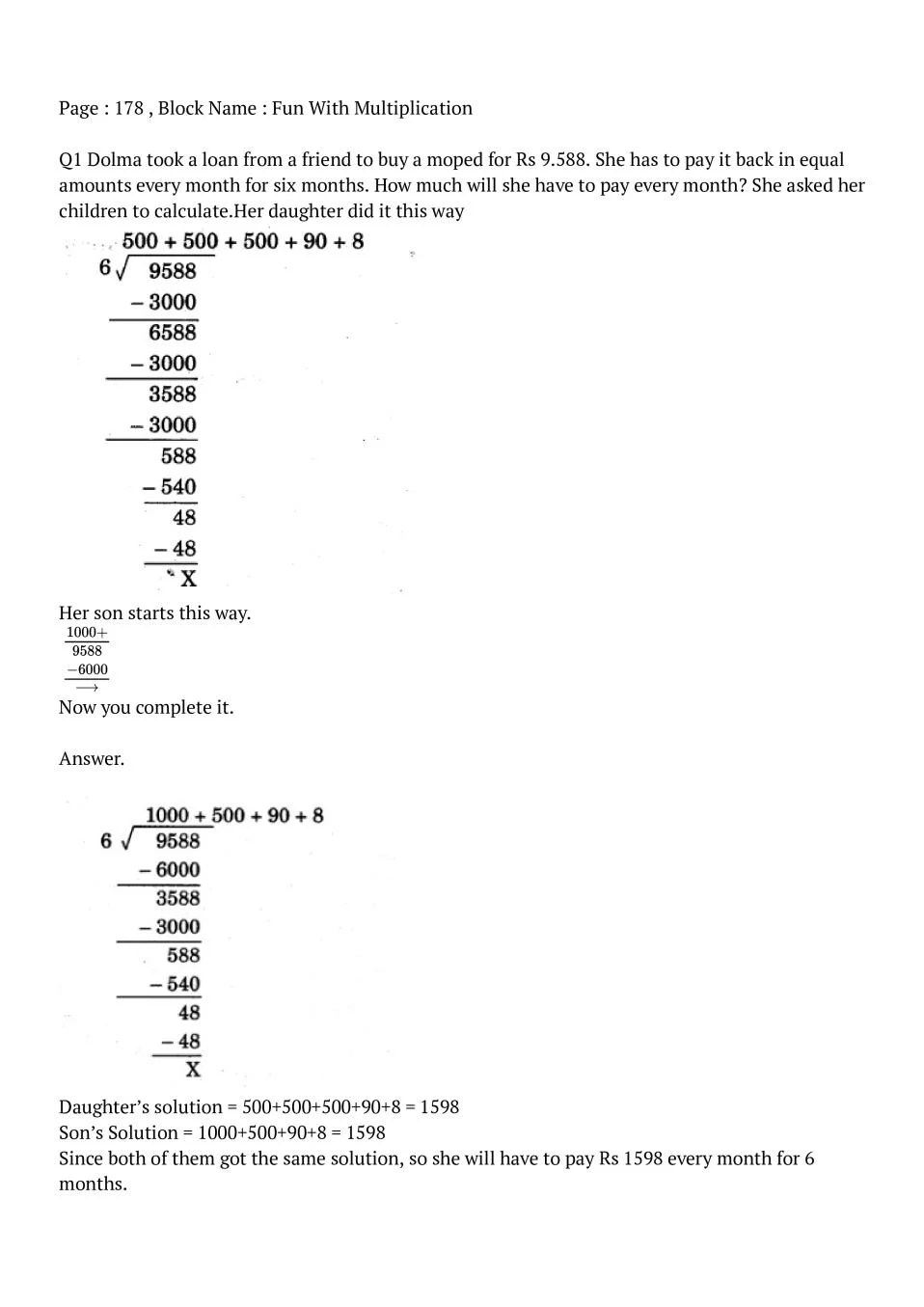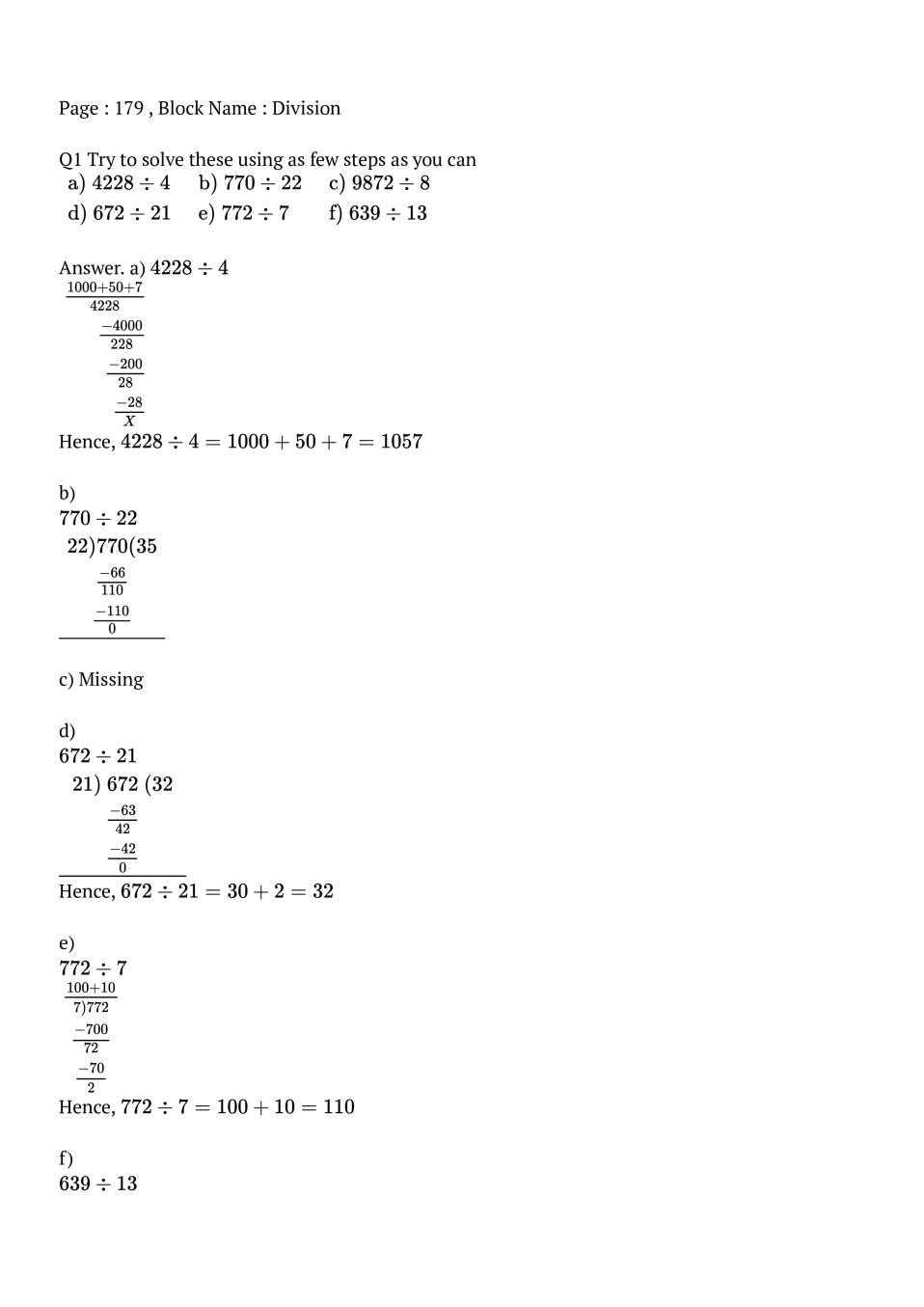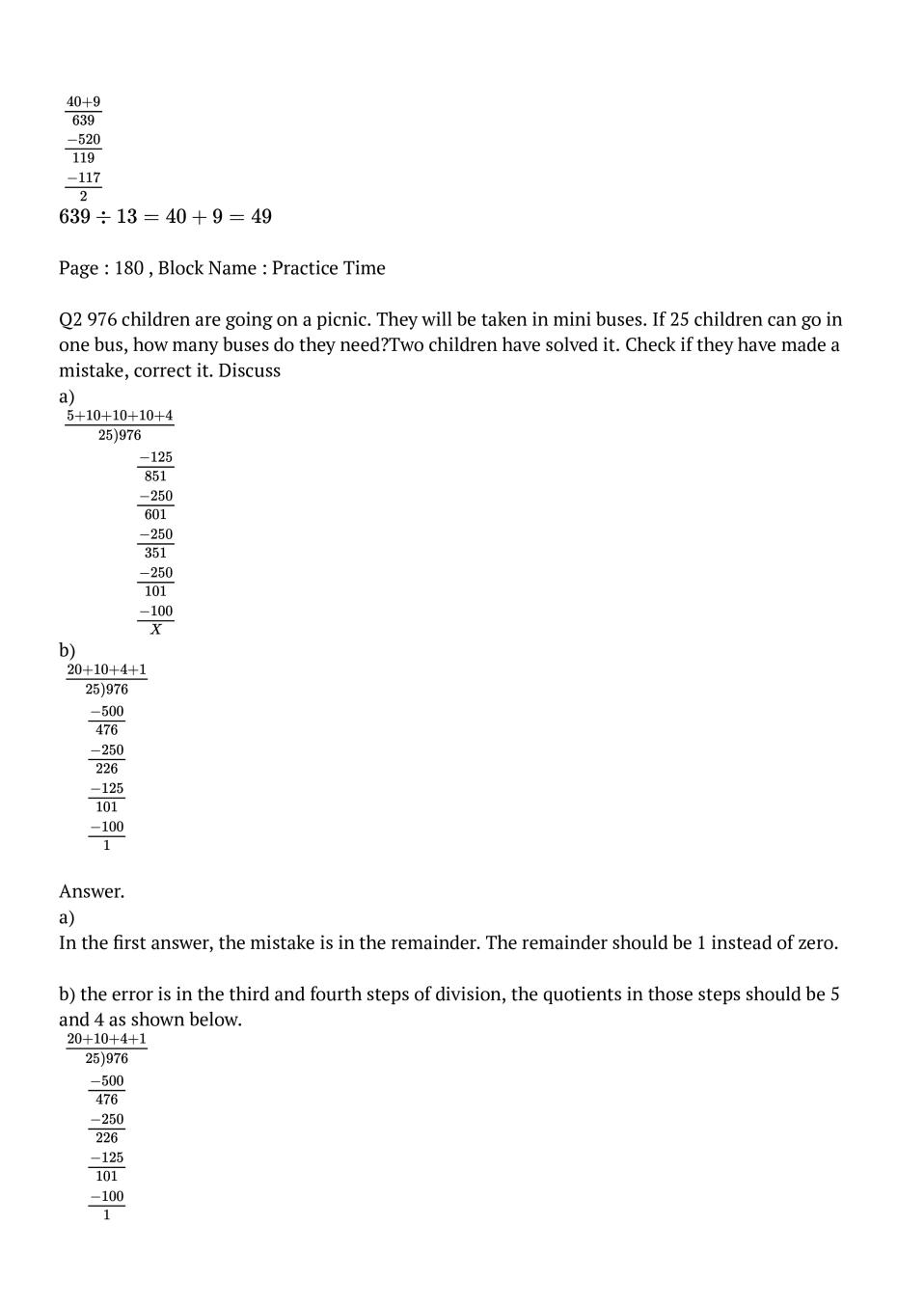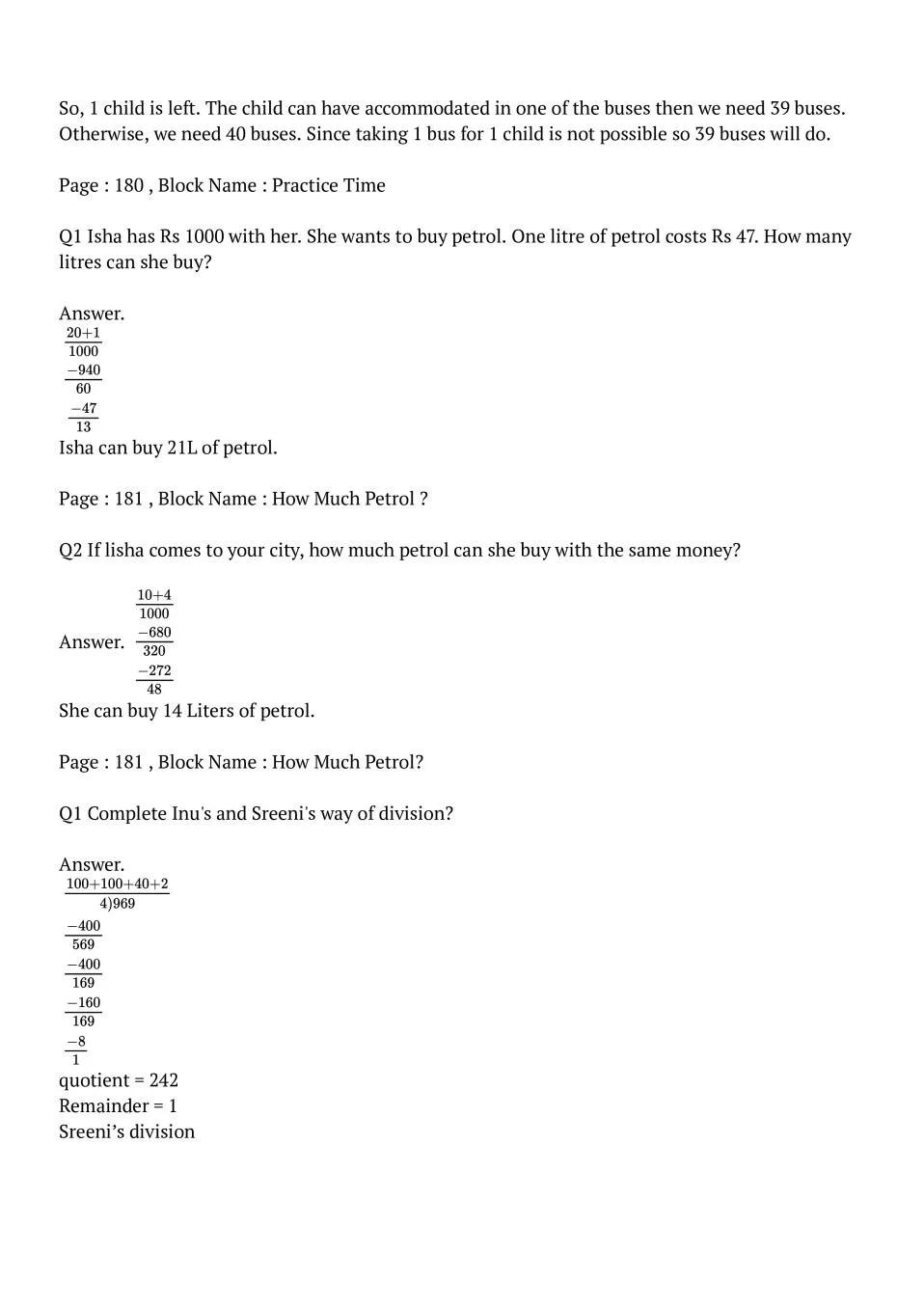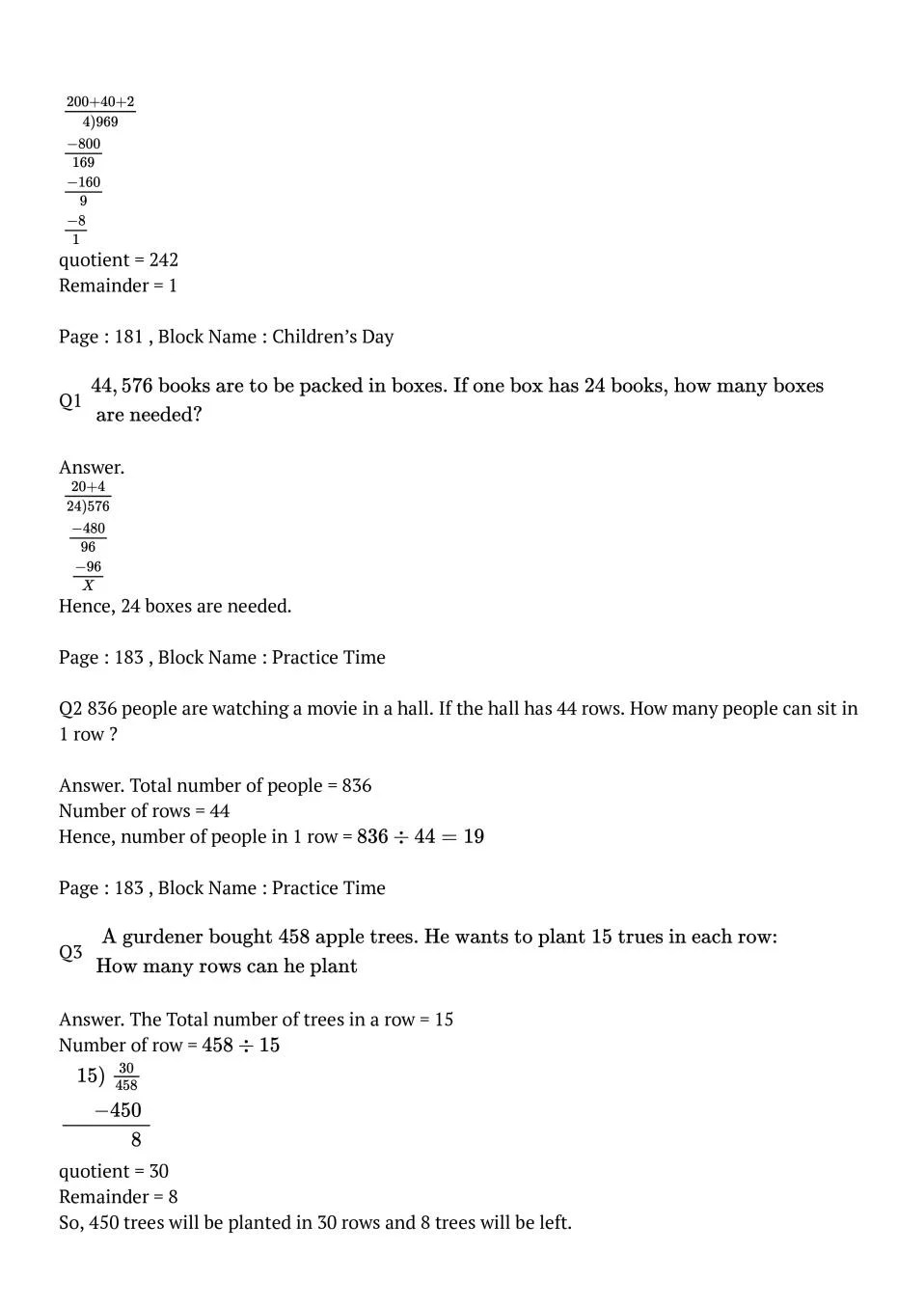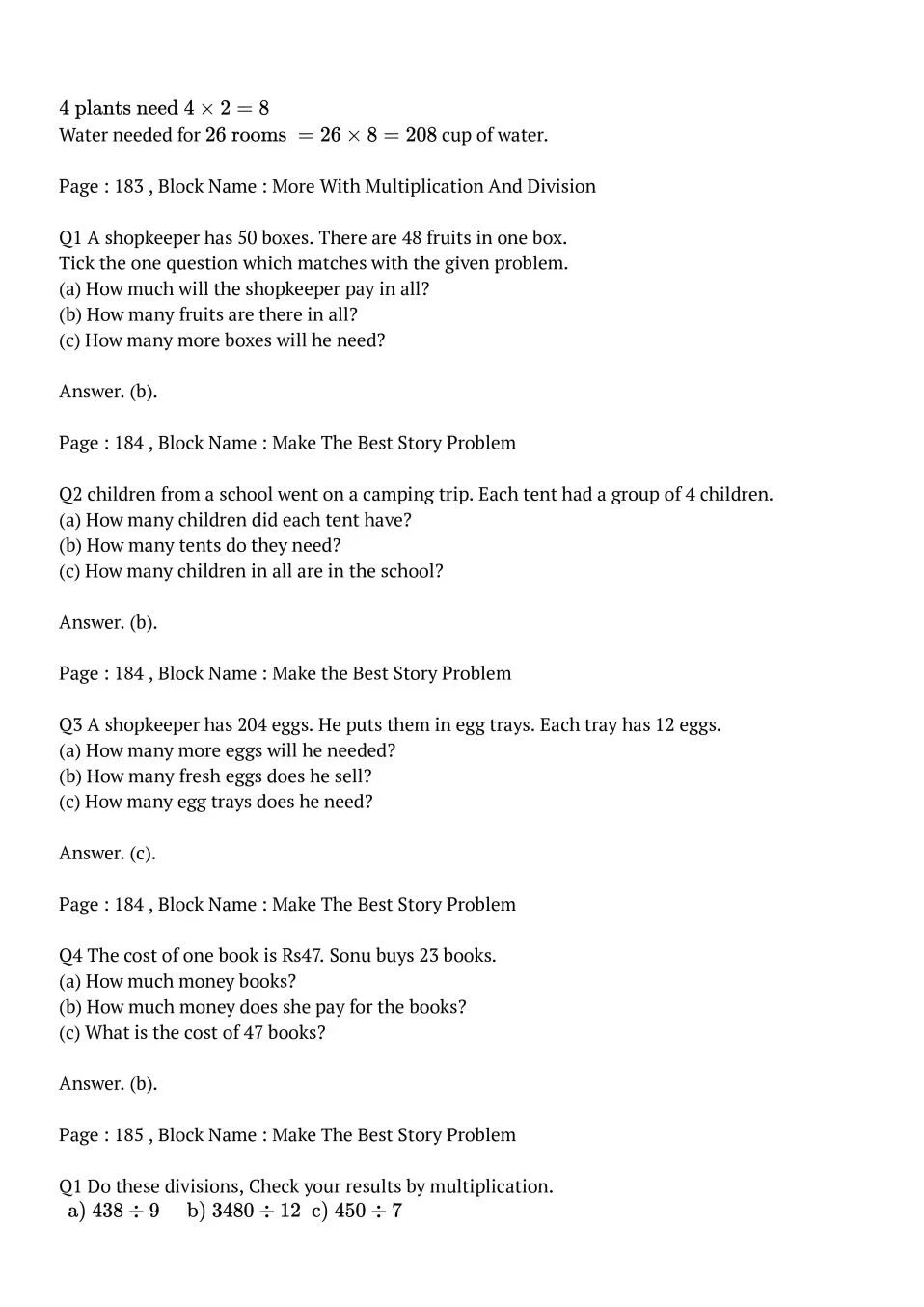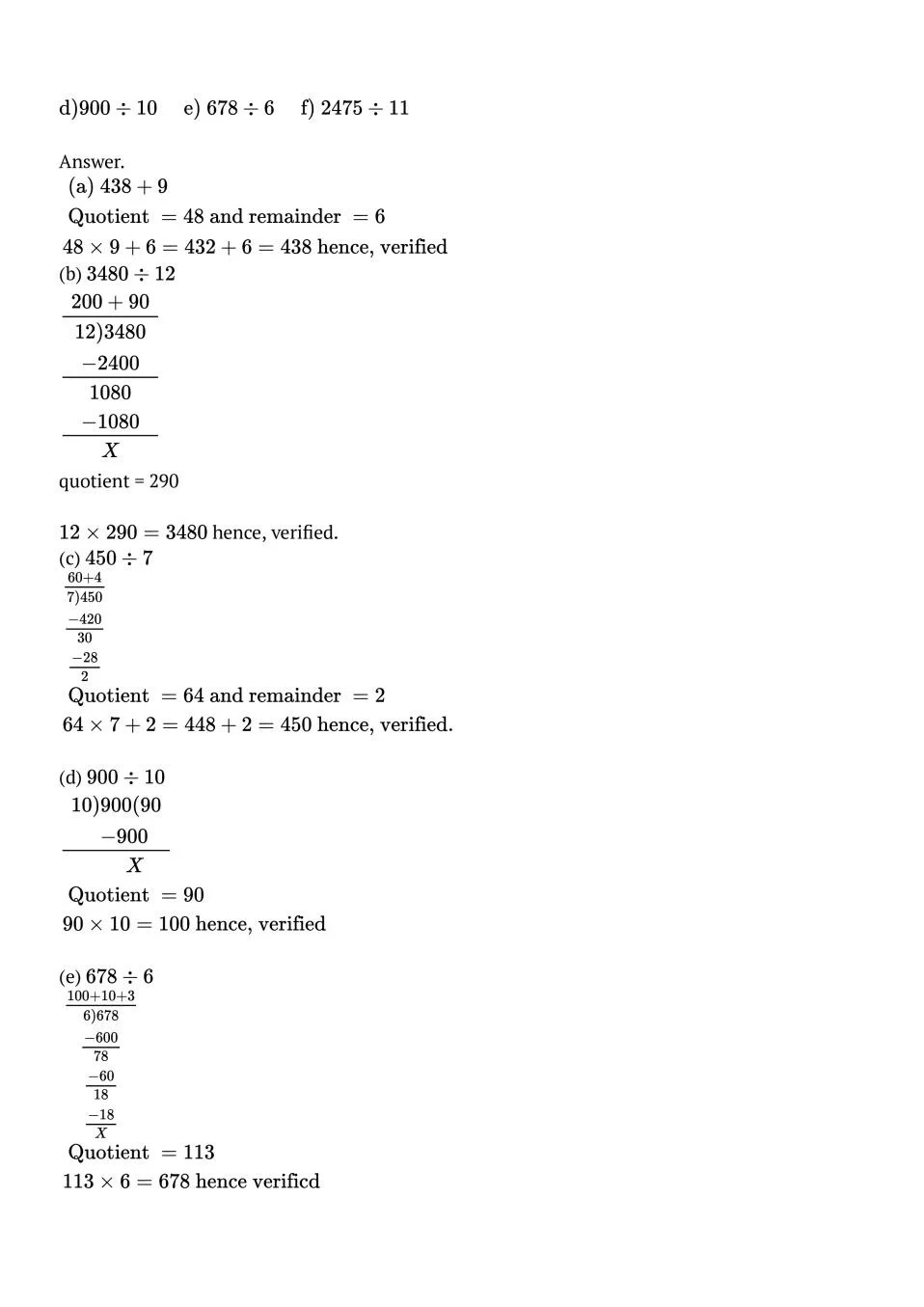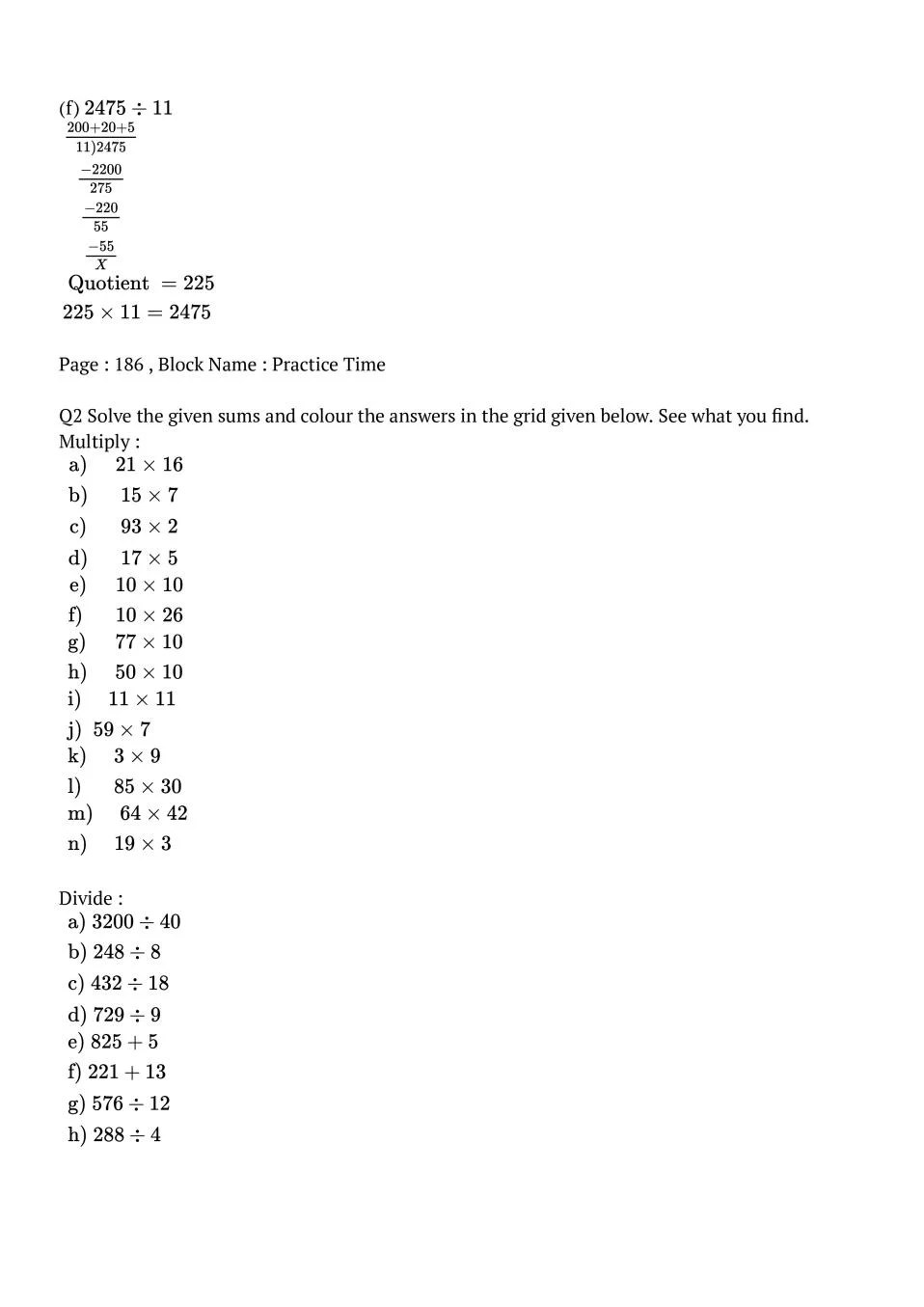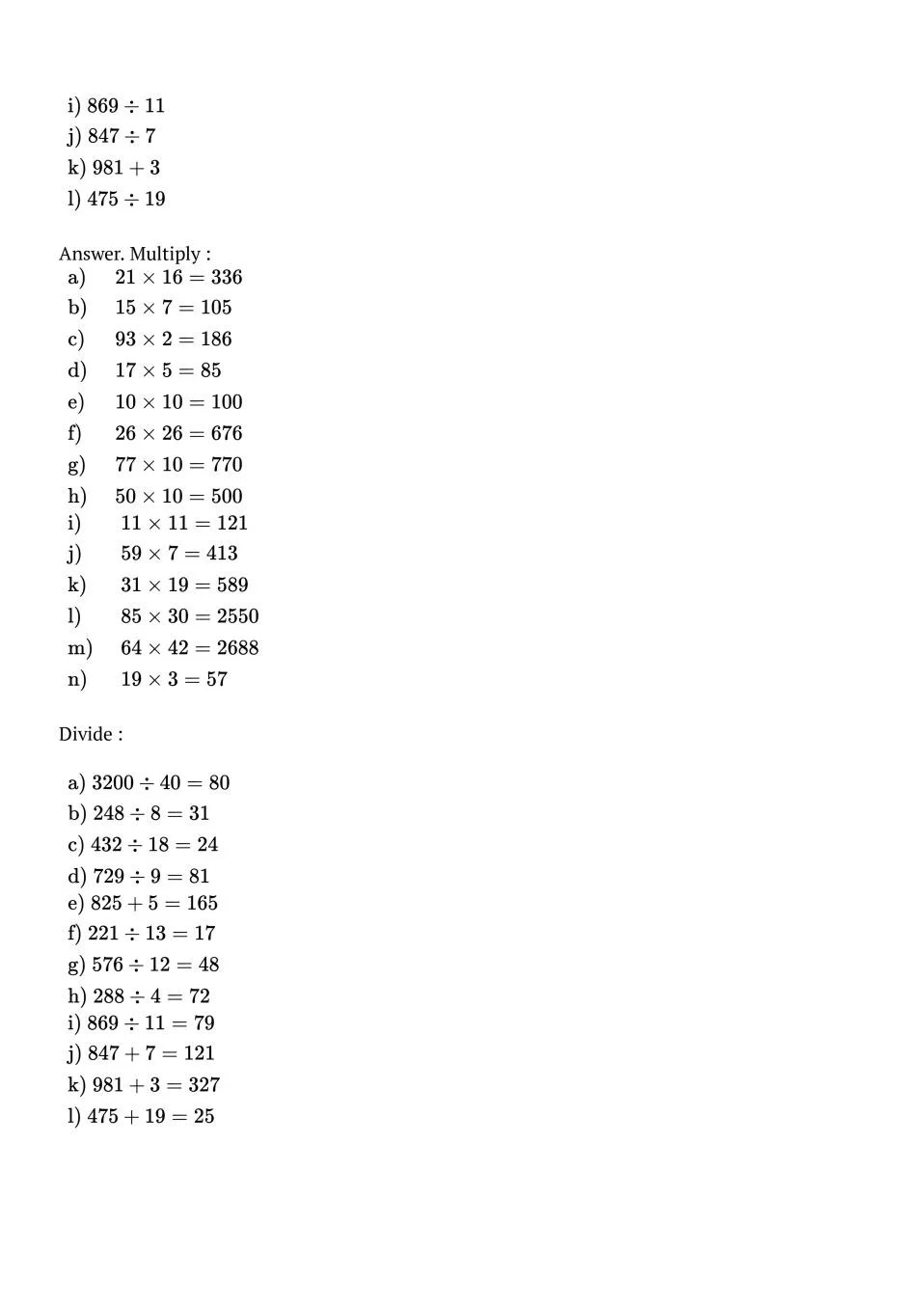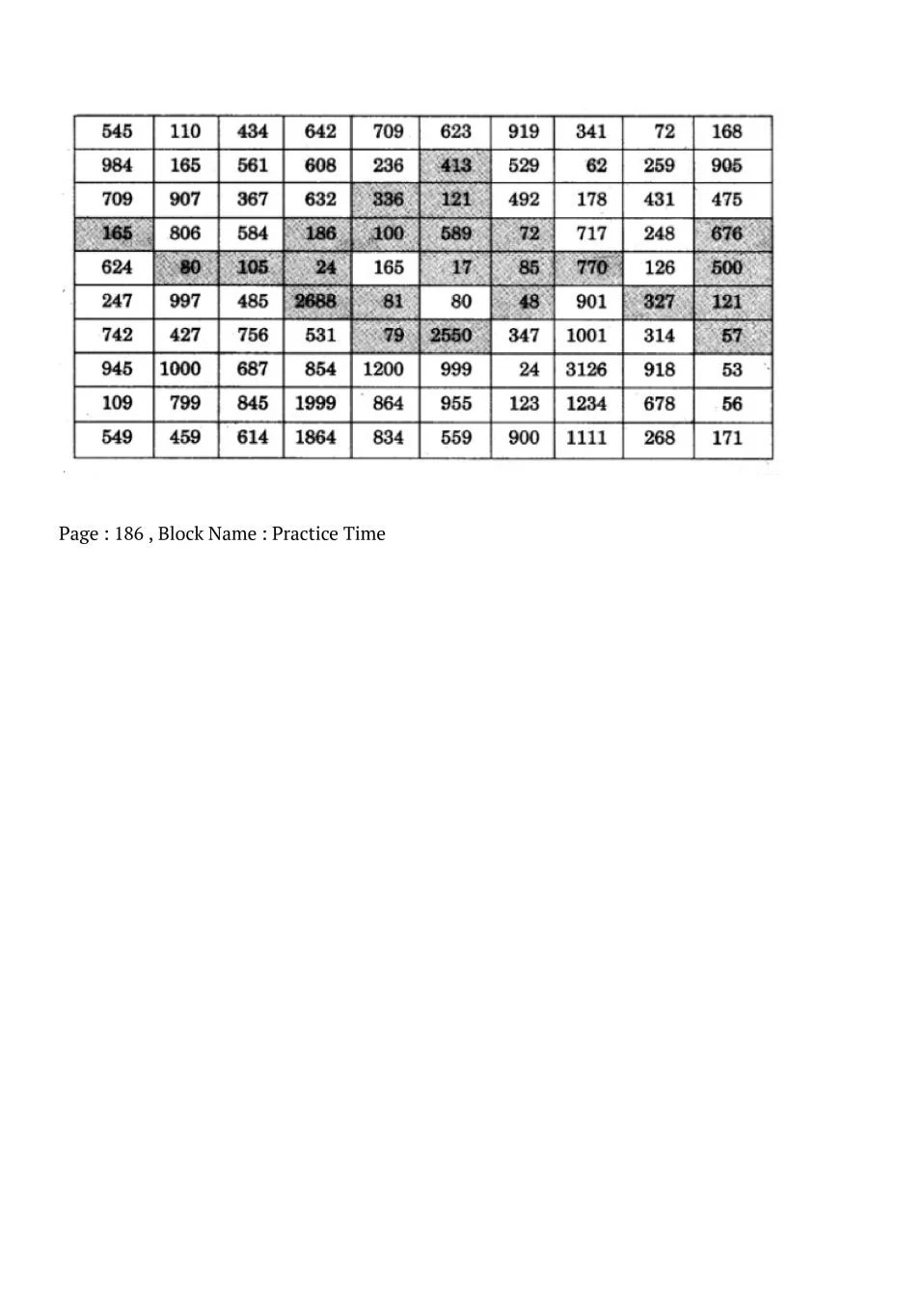### Class 5 Maths Chapter 13 NCERT Solutions

Get step by step solutions for the questions given in class 5 Maths textbook from the latest NCERT as per board exam guidelines. We also suggest you to practice all the solved examples given in the NCERT book to clear your concepts on Ways To Multiply And Divide. You can buy NCERT Books for Class 5 Maths online for doorstep delivery.

### NCERT Solutions Class 5 Chapter Wise

If you want NCERT solutions of any topic other than Chapter 13 Ways To Multiply And Divide, check it from here. Maths Book by NCERT has all chapters that are in your Class 5 syllabus.

### NCERT Solutions Class 5 All Subjects

All NCERT Solutions for Subjects other than English are provided below:

 English Hindi Maths EVS

### Other Classes NCERT Solutions

If you want NCERT solutions of any class other than Class 5, check it from here.